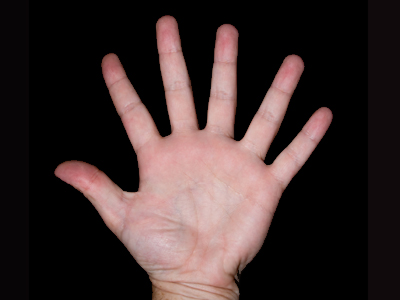6 is the digit in the ten thousands' place in 68,127.

# Place Value (Easy)

Place value is about the position of a number. In this 11-plus Maths quiz you will get the chance to revise place values in whole numbers < 100,000.

Use this information to help you find a digit's place value. Reading the number from right to left: the first digit is in the ones' place; the second digit is in the tens' place; the third digit is in the hundreds' place; the fourth digit is in the thousands' place; the fifth digit is in the ten thousands' place.

For example, in 83,659 the 9 is in the ones' place; the 5 is in the tens' place; the 6 is in the hundreds' place; the 3 is in the thousands' place; the 8 is in the ten thousands' place.

As you will probably gather, these quizzes get progressively harder so don't move on until you've got 10 out of 10 in this one!

1.
What is the place value of 2 in 92,347?
The hundreds' place
The thousands' place
The ten thousands' place
The tens' place
Reading the number from right to left: 2 is in the thousands' place
2.
What is the place value of 8 in 83,537?
The tens' place
The hundreds' place
The ten thousands' place
The thousands' place
Reading the number from right to left: 8 is in the ten thousands' place
3.
What is the digit in the thousands' place in 43,566?
5
4
3
6
Reading the number from right to left: 3 is in the thousands' place
4.
What is the digit in the tens' place in 63,507?
0
5
7
6
Reading the number from right to left: 0 is in the tens' place
5.
What is the place value of 5 in 63,597?
The ones' place
The thousands' place
The hundreds' place
The tens' place
Reading the number from right to left: 5 is in the hundreds' place
6.
What is the place value of 1 in 64,601?
The ones' place
The hundreds' place
The tens' place
The thousands' place
Reading the number from right to left: 1 is in the ones' place
7.
What is the place value of 3 in 63,400?
The hundreds' place
The thousands' place
The tens' place
The ten thousands' place
Reading the number from right to left: 3 is in the thousands' place
8.
What is the digit in the hundreds' place in 92,067?
9
6
7
0
Reading the number from right to left: 0 is in the hundreds' place
9.
What is the digit in the ten thousands' place in 68,127?
6
8
2
7
Reading the number from right to left: 6 is in the ten thousands' place
10.
What is the digit in the ones' place in 88,329?
3
2
8
9
Reading the number from right to left: 5 is in the ones' place
Author:  Frank Evans